# Examples of CUQI

Here we illustrate our work on computational UQ for inverse problems with a few examples from ongoing projects at DTU Compute. These examples illustrate various methodologies for implementing, applying and using UQ.

Tikhonov Regularization with Nonnegativity Constraints

Johnathan M. Bardsley, Univ. of Montana and Per Christian Hansen

Nonnegativity constraints appear in many applications where the underlying physics dictates that the solution cannot be negative. This is the case, e.g., for absorption coefficients in computed tomography, image intensities in astronomical imaging, and wave velocities in seismic travel-time tomography. Hence, nonnegativity constraints are very important for producing meaningful reconstructions,

We considers computational aspects of UQ for Tikhonov regularization problems with nonnegativity constraints, formulated in a Bayesian setting:

minx || A x – b ||22 + λ || x ||22   subject to   x ≥ 0 .

If we replace the nonnegativity constraint with a positivity constraint, x > 0, then we can handle this constraint within the "standard" UQ framework with a transformation of variables x = exp(z). But in this work we specifically address situations where we expect the existence of zero elements in the solution – in fact, in some imaging problems a substantial fraction of the solution's elements/pixels may be zero. Therefore we choose to use a nonnegative Gaussian distribution.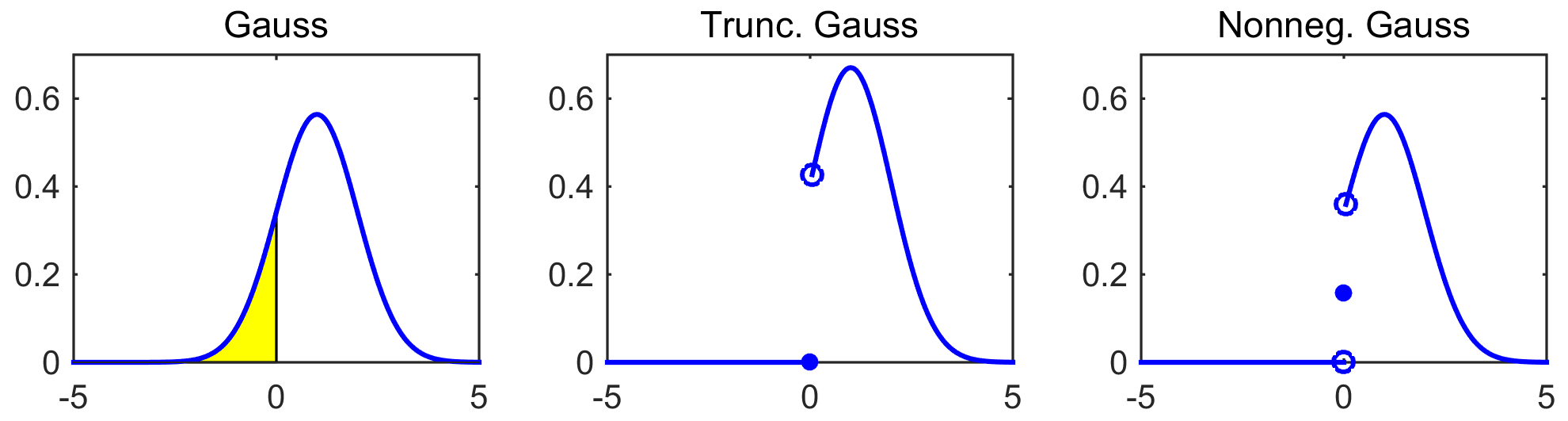The above figure shows a simple Gauss distribution (left), a truncated Gauss distribution with zero probability for x = 0 (middle), and a nonnegative Gaussian distribution with nonzero probability for x = 0 (right).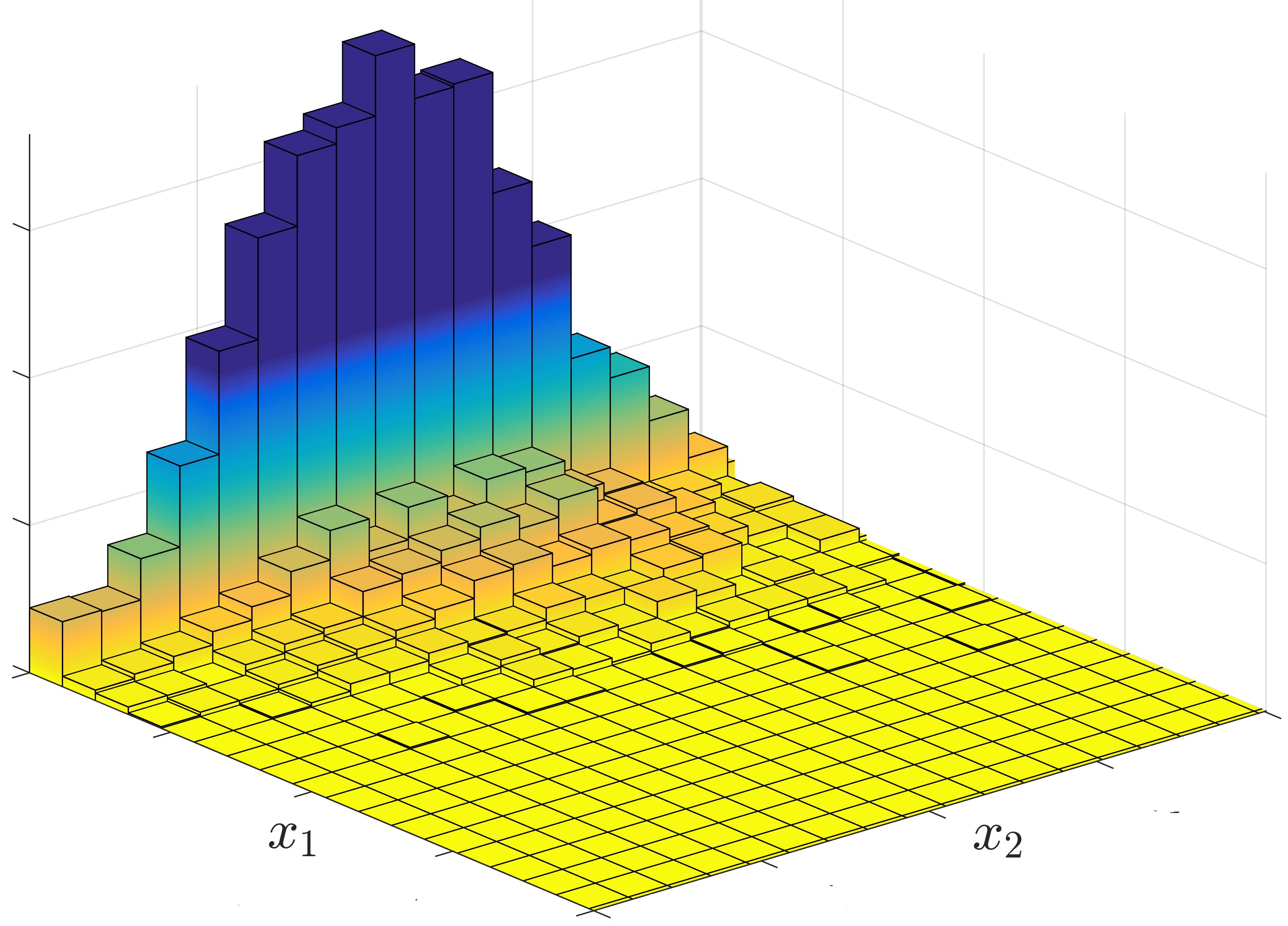This figure shows a histogram of a simple 2D nonnegativity constrained least squares problem (i.e., α = 0) whose exact solution is (x1,x2) = (0,1), associated with a 2D nonnegative Gauss distribution. Notice the significant "mass" of the histogram for x1 = 0. Except for very simple cases the corresponding probability density function for the posterior does not have a convenient analytical expression. Instead we use a Markov chain Monte Carlo (MCMC) method to sample the constrained regualrized solution's probability distribution, and we refer to our algorithm as a Nonnegative Hierarchical Gibbs Sampler.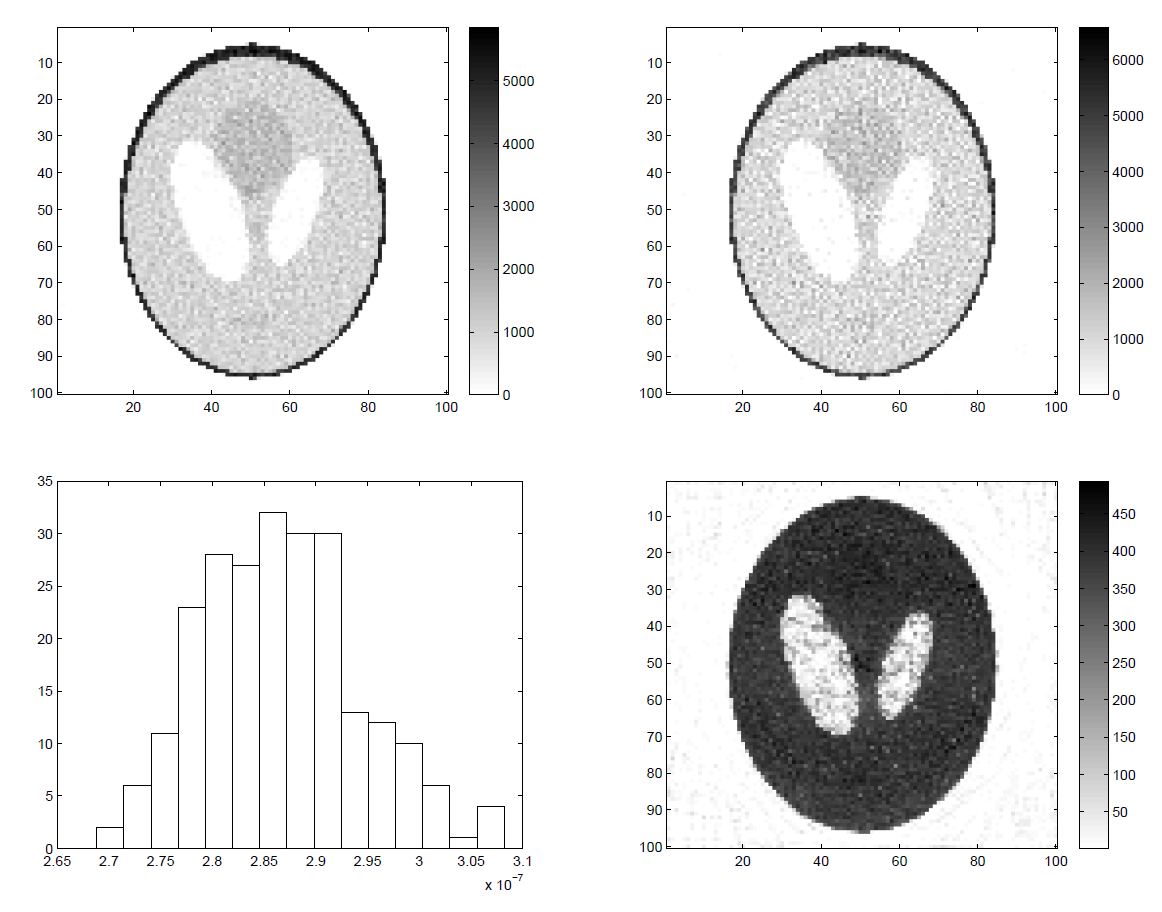We applied our algorithm to positron emission tomography (PET), where a radioactive tracer element is injected into a body and exhibits radioactive decay, resulting in photon emission. The emitted photons that leave the body are recorded by a photon detector, and the resulting mathematical reconstruction problem is equivalent to a classical X-ray CT problem. The above figure shows our simulation results.

• Top left: the mean of the computed image samples from our nonnegative hierarchical Gibbs sampler.
• Bottom left: histogram of the regularization parameters produced by the sampler.
• Top right: the MAP estimate using the mean of the regularization parameters.
• The pixel-wise standard deviations from the sampler. As expected, image pixels with higher intensity also have higher variance.

For details see: J. M. Bardsley and P. C. Hansen, MCMC algorithms for computational UQ of nonnegativity constrained linear inverse problems, in review.

## One-Dimensional EIT with a Stochastic Differential Equation

Mirza Karamehmedović

This example illustrates UQ applied to a stochastic model of a physical phenomenon. Specifically, we consider a stochastic differential equation that models a simple electric direct-current problem which can be considered a "1D electrical impedance tomography (EIT) problem."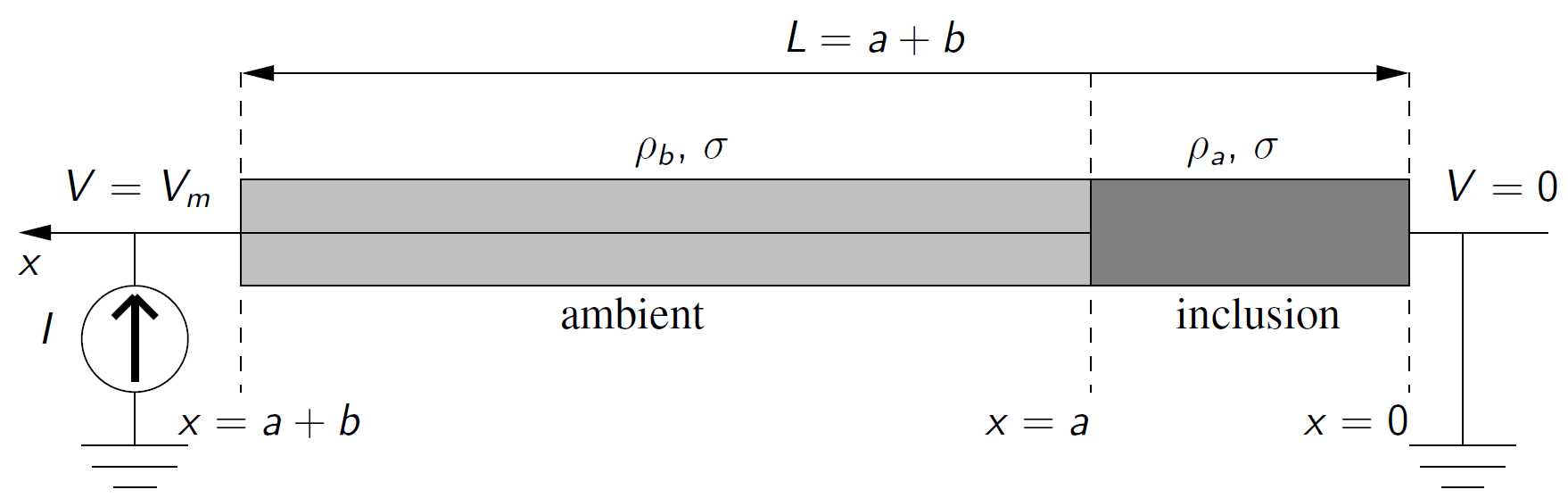The expected resistivities ρa and ρof the inclusion and the ambient are assumed to be known. The length a of the inclusion is estimated in terms of a probability density, based on measurements of potential Vm at x = a+b. In each of the intervals 0 < x < a and a < x < b, the stochastic differential equation that describes the change of the potential V as function of the displacement x is

dVx = I ρ dx + σ dWx .

Here the ambient resistivity ρ is the constant ρa or ρb, depending on the considered interval. The constant σ is the same in the two intervals for x.  Moreover, dWx is a white-noise term that introduces the stochasticity. Through the use of a forward Fokker-Planck equation, we show that the corresponding model sampling density is

p(Vm | a) = (2π σ2 L)-1/2 exp(  (Vm  I (a ρa + (La) ρb))2 / (2 σ2 L),      a ∈ [0,L] ,

and then Bayes' theorem leads to the posterior

p(a | Vm) = const ⋅ p(Vm | a) p(a) .

Here, Vm is the measured data, a is the model parameter, p(| Vm) is the posterior distribution for the parameter a given the measurement Vm, and p(Vm | a) is the sampling density for the data. Finally, p(a) is the prior distribution for the parameter a.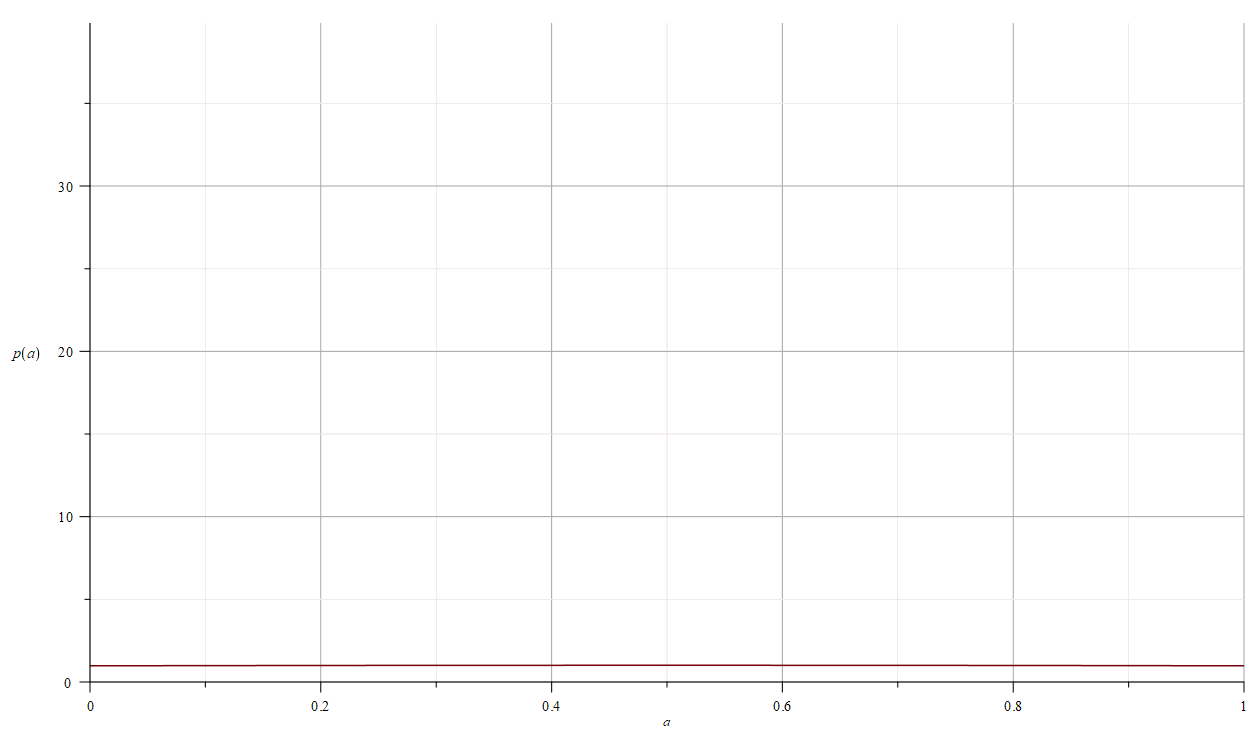The above animation shows the evolution of the posterior p(a | Vm) as we obtain an increasing number of observations.  In this example the prior p(a)  is a truncated normal distribution over [0,L].

This is ongoing work at DTU Compute.

## Handling Uncertain Projection Angles in CT via a Model Discrepancy

Nicolai A. B. Riis, Yiqiu Dong, and Per Christian Hansen

This example illustrates the use of UQ in a case where the forward model has uncertain model-parameters. Specifically we consider a Computed Tomography (CT) problem where the projection angles are not known precisely - but we have a statistical model for them.

Our goal is to compute the unknown attenuation coefficient image, represented by the vector x, from measured and noisy data represented by the vector b. This is done according to the model

b = R(θ) x + e ,     θπangles(⋅) ,     eπnoise(⋅) ,

where the matrix R(θ) represents the discretized Radon transform for the projection angles θ, and the uncertainty in the angles and the data are characterized by the probability distributions πangles and πnoise, respectively. We fix the forward model with a single estimate θo of the projection angles which represent our belief of the actual angles in the scanner. We then obtain the model

b = R(θo) x + d + e ,      eπnoise(⋅) ,

where the model discrepancy term d acquires its push-forward distribution from

d = d(θ,x) = R(θ) xR(θo) x .

Inspired by work by Kaipio & Somersalo we marginalize both d and e, to arrive at the following likelihood for b:

π(b|x) = πν|x(b  R(θo) x | x) ,     ν = d + e .

We now assume that both d|x and e follow Gaussian distributions, and hence πν|x = 𝒩(μ,C). Then the likelihood has a closed-form expression, and taking the negative logarithm we obtain

log π(b|x) ∝ || L (b  R(θo) x  μ) ||22 ,

where LTL = C1 is the Cholesky factorization of the inverse covariance matrix. Adding Total Variation (TV) regularization and a nonnegativity constraint we arrive at the variational model

minx || L (bR(θo) x  μ) ||22 + λTV(x)   subject to   x ≥ 0.

We developed a new iterative algorithm MD-TV that alternates between updating the solution x and the pair (μ,C). The latter is achieved by computing samples of d|x, and we utilize block structure in the computational problem for efficiency.The above figure compares reconstructions for two choices of the regularization parameter λ from simulation studies.  From left to right:

• TV denotes a standard TV algorithm that ignores the uncertainty in the projection angles and uses the assumed angles θo. These reconstructions are noisy due to the uncertain projection angles.
• MV-TV denotes our new algorithm which produces improved reconstructions with less noise.
• TV-opt denotes TV reconstructions based on the true - but unknown - projection angles; this defines the unattainable optimal reconstruction from the noisy data.

For details see: N. A. B. Riis, Y. Dong, and P. C. Hansen, Computed tomography reconstruction with uncertain view angles by iteratively updated model discrepancy, submitted.

https://www.compute.dtu.dk/english/cuqi/examples-of-cuqi
14 APRIL 2021